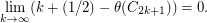# Shannon capacity of the seven-cycle

 Importance: High ✭✭✭
 Author(s):
 Subject: Graph Theory
 Keywords:
Problem   What is the Shannon capacity of?
Letdenote the independence number of the graph, and letdenote the strong graph product ofand(in which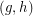is adjacent toif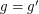andis adjacent to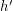, or if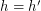and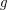is adjacent to, or ifis adjacent toandis adjacent to). Then the Shannon capacity ofis defined by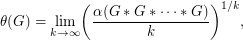where the strong graph product is overcopies of. The Shannon capacity is important because it represents the effective size of an alphabet in a communication model represented by, but it is notoriously difficult to compute. Lovász [L] famously proved that the Shannon capacity of the five-cycleis, but even the Shannon capacity ofremains unknown. However, Bohman [B] has shown that